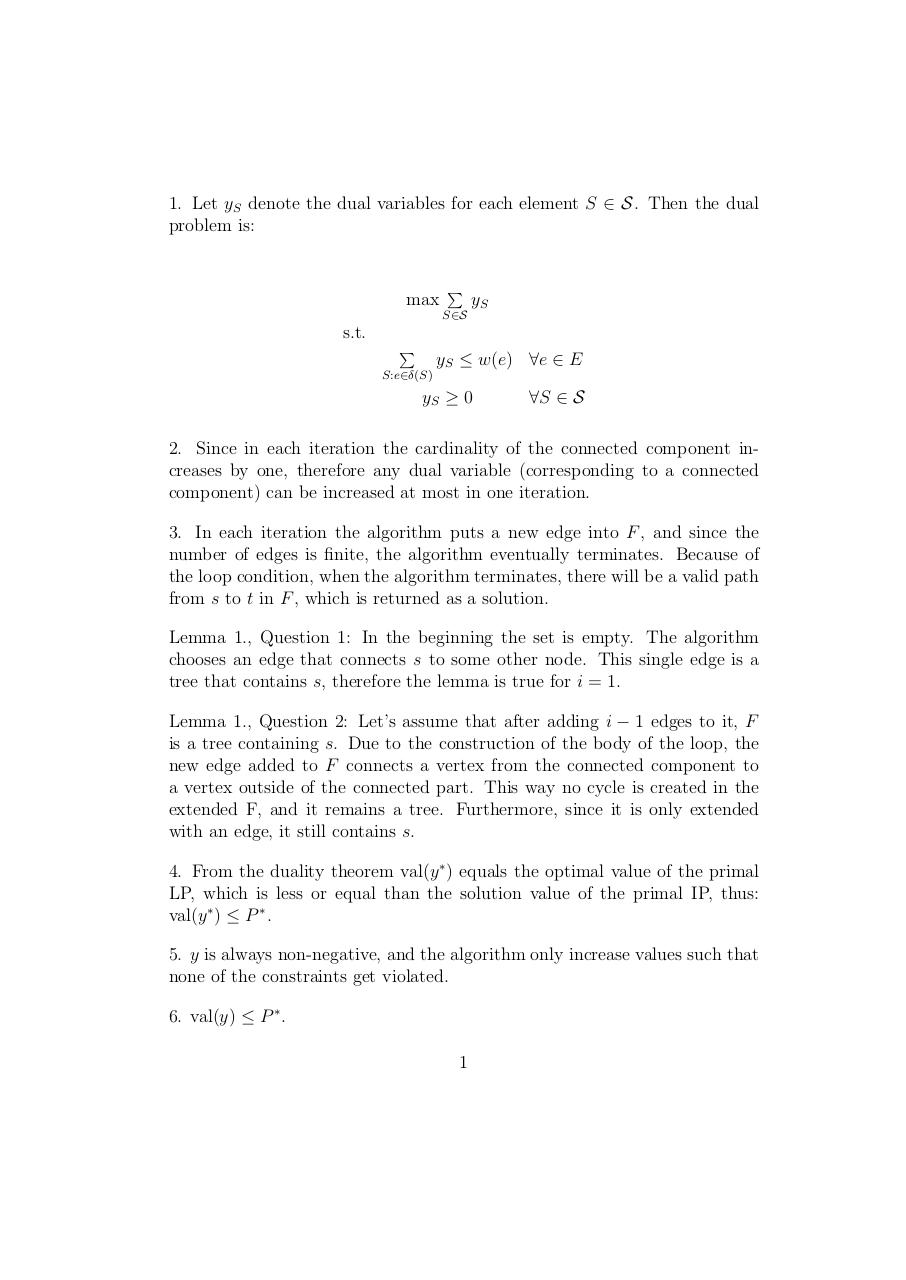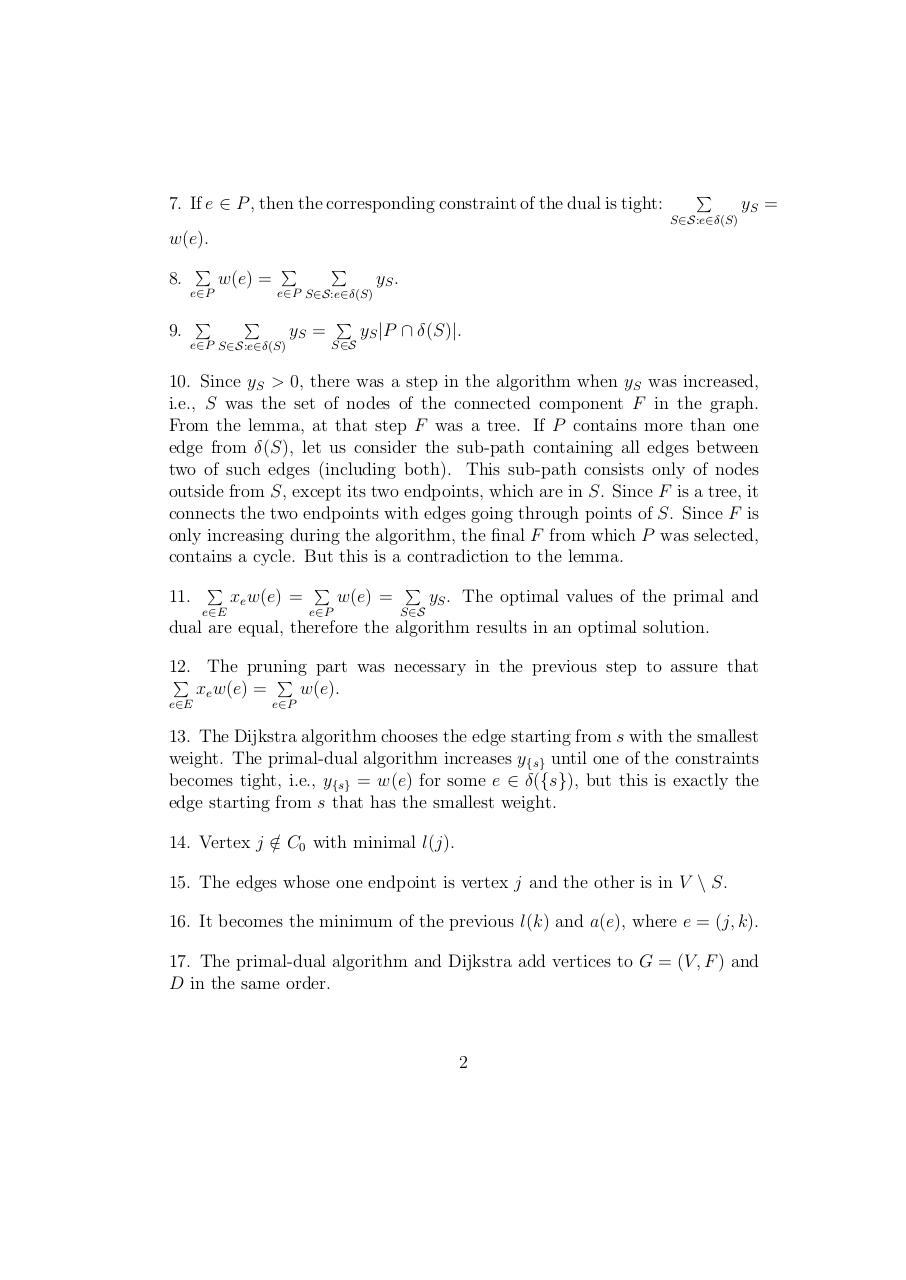# shortestpath .pdf

### File information

Original filename: shortestpath.pdf

This PDF 1.5 document has been generated by TeX / pdfTeX-1.40.14, and has been sent on pdf-archive.com on 12/02/2016 at 10:13, from IP address 195.111.x.x. The current document download page has been viewed 414 times.
File size: 75 KB (3 pages).
Privacy: public file

shortestpath.pdf (PDF, 75 KB)

### Document preview

1. Let yS denote the dual variables for each element S ∈ S. Then the dual
problem is:

max

P

yS

S∈S

s.t.
yS ≤ w(e) ∀e ∈ E

P
S:e∈δ(S)

yS ≥ 0

∀S ∈ S

2. Since in each iteration the cardinality of the connected component increases by one, therefore any dual variable (corresponding to a connected
component) can be increased at most in one iteration.
3. In each iteration the algorithm puts a new edge into F , and since the
number of edges is finite, the algorithm eventually terminates. Because of
the loop condition, when the algorithm terminates, there will be a valid path
from s to t in F , which is returned as a solution.
Lemma 1., Question 1: In the beginning the set is empty. The algorithm
chooses an edge that connects s to some other node. This single edge is a
tree that contains s, therefore the lemma is true for i = 1.
Lemma 1., Question 2: Let’s assume that after adding i − 1 edges to it, F
is a tree containing s. Due to the construction of the body of the loop, the
new edge added to F connects a vertex from the connected component to
a vertex outside of the connected part. This way no cycle is created in the
extended F, and it remains a tree. Furthermore, since it is only extended
with an edge, it still contains s.
4. From the duality theorem val(y ∗ ) equals the optimal value of the primal
LP, which is less or equal than the solution value of the primal IP, thus:
val(y ∗ ) ≤ P ∗ .
5. y is always non-negative, and the algorithm only increase values such that
none of the constraints get violated.
6. val(y) ≤ P ∗ .
1

7. If e ∈ P , then the corresponding constraint of the dual is tight:

P

yS =

S∈S:e∈δ(S)

w(e).
P

8.

w(e) =

e∈P

P

9.

P

P

yS .

e∈P S∈S:e∈δ(S)

P

P

yS =

e∈P S∈S:e∈δ(S)

yS |P ∩ δ(S)|.

S∈S

10. Since yS &gt; 0, there was a step in the algorithm when yS was increased,
i.e., S was the set of nodes of the connected component F in the graph.
From the lemma, at that step F was a tree. If P contains more than one
edge from δ(S), let us consider the sub-path containing all edges between
two of such edges (including both). This sub-path consists only of nodes
outside from S, except its two endpoints, which are in S. Since F is a tree, it
connects the two endpoints with edges going through points of S. Since F is
only increasing during the algorithm, the final F from which P was selected,
contains a cycle. But this is a contradiction to the lemma.
11.

P

xe w(e) =

e∈E

P
e∈P

w(e) =

P

yS . The optimal values of the primal and

S∈S

dual are equal, therefore the algorithm results in an optimal solution.
12. The pruning part was necessary in the previous step to assure that
P
P
xe w(e) =
w(e).
e∈E

e∈P

13. The Dijkstra algorithm chooses the edge starting from s with the smallest
weight. The primal-dual algorithm increases y{s} until one of the constraints
becomes tight, i.e., y{s} = w(e) for some e ∈ δ({s}), but this is exactly the
edge starting from s that has the smallest weight.
14. Vertex j ∈
/ C0 with minimal l(j).
15. The edges whose one endpoint is vertex j and the other is in V \ S.
16. It becomes the minimum of the previous l(k) and a(e), where e = (j, k).
17. The primal-dual algorithm and Dijkstra add vertices to G = (V, F ) and
D in the same order.

2

18. The worst-case complexity of the primal-dual algorithm is O(|E| + |V |2 ).

3#### HTML Code

Copy the following HTML code to share your document on a Website or Blog

#### QR Code### Related keywords